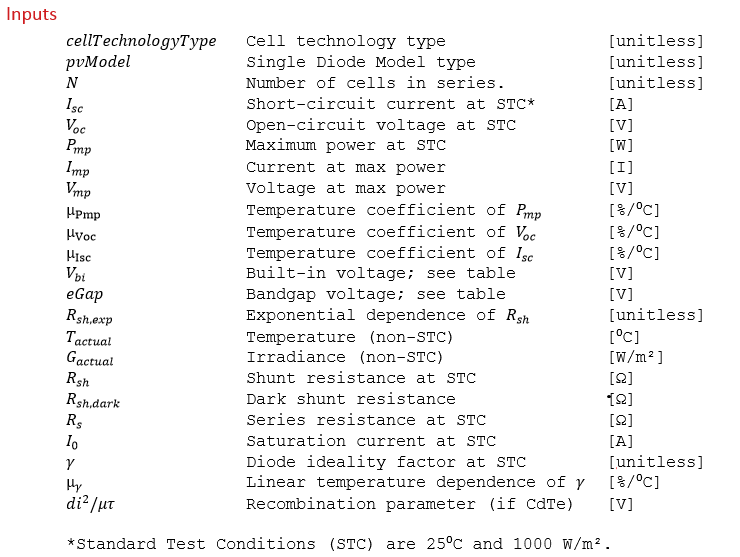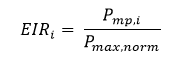# Effective Irradiance Response (EIR) Calculation

The effective irradiance response (EIR) of a module is its relative efficiency at various non-standard conditions. This is sometimes referred to as a module’s “low-light response”. The following algorithm describes how to calculate the relative efficiency at a single condition (non-STC temperature/irradiance). This process can be repeated at various conditions to generate an EIR curve.1. Calculate the maximum power point at Standard Test Conditions (STC).

The maximum power point is calculated using single diode parameters at STC.

1. Temperature and irradiance adjust all pertinent single diode parameters to T_actual and G_actual.

See the algorithm documentation section entitled “One-Diode Model Temperature Correction”.

1. Calculate the maximum power point from the temperature/irradiance-adjusted single diode parameters.

Using the temperature-adjusted single diode parameters, calculate the maximum power point as in step 1.

1. Calculate the relative efficiency.where EIRi is the relative efficiency at a given irradiance in decimal form,  Pmp,i is the maximum power point at a given irradiance, and Pmax,norm is the normalized maximum (ideal) power point at a given irradiance, as described by the following equation:where Pmp,1000 is the maximum power point at 1000 W/m2 and i is the given irradiance.